# Control hardware: pulse calibration

Calibrating pulses for maximum performance on your hardware

In this Application note, you'll learn all the steps required to calibrate your pulses, illustrated with examples on how to implement these techniques using IBM-Quantum hardware.

Calibration is the first step to ensure that the pulses you apply on your qubits perform the desired operations. This is true for any pulse, from a simple square waveform to more complex time-dependent controls, as the calibration corrects for discrepancies between the pulse that you programmed in your waveform generator and what actually reaches the qubit.

In the first section of this notebook, you'll learn how to map the output pulse amplitudes from BOULDER OPAL into hardware inputs. In the second section, you'll be presented with a fine-tuning calibration procedure for the $I$ and $Q$ values of a given pulse to maximize the gate fidelity. In the final section you'll use BOULDER OPAL's closed-loop optimization tools to perform the calibration in an automated and more efficient way.

Some cells in this notebook require an account with IBM-Q to execute correctly. If you want to run them, please go to the IBM-Q experience to set up an account.

## Imports and initialization

import jsonpickle
import matplotlib.gridspec as gridspec
import matplotlib as mpl
import matplotlib.pyplot as plt
from mpl_toolkits.mplot3d import Axes3D
from matplotlib import cm
from matplotlib.ticker import LinearLocator, FormatStrFormatter
from matplotlib import colors

import numpy as np
from qctrlvisualizer import get_qctrl_style, plot_controls
from scipy import interpolate
from scipy.optimize import curve_fit

# Q-CTRL imports
from qctrl import Qctrl

# Starting a session with the API
qctrl = Qctrl()

# Choose to run experiments or to use saved data
use_saved_data = True

# Plotting parameters
plt.style.use(get_qctrl_style())
# prop_cycle = plt.rcParams["axes.prop_cycle"]
# colors = prop_cycle.by_key()["color"]
markers = {"x": "x", "y": "s", "z": "o"}
lines = {"x": "--", "y": "-.", "z": "-"}

# Definition of operators and functions
sigma_z = np.array([[1.0, 0.0], [0.0, -1.0]], dtype=complex)
sigma_x = np.array([[0.0, 1.0], [1.0, 0.0]], dtype=complex)
sigma_y = np.array([[0.0, -1.0j], [1.0j, 0.0]], dtype=complex)
X90_gate = np.array([[1.0, -1j], [-1j, 1.0]], dtype=complex) / np.sqrt(2)
bloch_basis = ["x", "y", "z"]

def save_var(file_name, var):
# saves a single var to a file using jsonpickle
f = open(file_name, "w+")
to_write = jsonpickle.encode(var)
f.write(to_write)
f.close()

def load_var(file_name):
# retuns a var from a json file
f = open(file_name, "r+")
encoded = f.read()
decoded = jsonpickle.decode(encoded)
f.close()
return decoded

def fit_function_bounds(x_values, y_values, function, bound_values):
fitparams, conv = curve_fit(function, x_values, y_values, bounds=bound_values)
y_fit = function(x_values, *fitparams)
return fitparams, y_fit

def movingaverage(x, w):
return np.convolve(x, np.ones(w), "valid") / w

def simulation_coherent(control, time_samples):

durations = [segment["duration"] for segment in control["I"]]
I_values = np.array([segment["value"] for segment in control["I"]])
Q_values = np.array([segment["value"] for segment in control["Q"]])
duration = sum(durations)

# Define shift controls
shift_I = qctrl.types.coherent_simulation.Shift(
control=[
qctrl.types.RealSegmentInput(duration=d, value=v)
for d, v in zip(durations, I_values)
],
operator=sigma_x,
)
shift_Q = qctrl.types.coherent_simulation.Shift(
control=[
qctrl.types.RealSegmentInput(duration=d, value=v)
for d, v in zip(durations, Q_values)
],
operator=sigma_y,
)

# Define sample times for the output
sample_times = np.linspace(0, duration, time_samples)

# Define the target (optional)
target = qctrl.types.TargetInput(operator=X90_gate)

# Perform simulation
simulation_result = qctrl.functions.calculate_coherent_simulation(
duration=duration,
sample_times=sample_times,
shifts=[shift_I, shift_Q],
initial_state_vector=np.array([1.0, 0.0]),
target=target,
)

# Extract results
gate_times = np.array([sample.time for sample in simulation_result.samples])
state_vectors = np.array(
[sample.state_vector for sample in simulation_result.samples]
)
infidelities = np.array([sample.infidelity for sample in simulation_result.samples])

bloch_vector_components = {
"x": np.real(
np.array(
[
np.linalg.multi_dot([np.conj(state), sigma_x, state])
for state in state_vectors
]
)
),
"y": np.real(
np.array(
[
np.linalg.multi_dot([np.conj(state), sigma_y, state])
for state in state_vectors
]
)
),
"z": np.real(
np.array(
[
np.linalg.multi_dot([np.conj(state), sigma_z, state])
for state in state_vectors
]
)
),
}

return infidelities, bloch_vector_components, gate_times

import warnings

warnings.simplefilter("ignore")
use_IBM = False

if use_IBM == True:
# IBM-Q imports
import qiskit as qs
import qiskit.pulse as pulse
from qiskit.pulse.library import Waveform
from qiskit import IBMQ, execute
from qiskit.compiler import assemble
from qiskit.pulse import Acquire, Play, Schedule
from qiskit.tools.jupyter import *
from qiskit.tools.monitor import job_monitor
from qiskit.ignis.verification import marginal_counts
from qiskit.ignis.mitigation.measurement import (
complete_meas_cal,
CompleteMeasFitter,
)

# IBM credentials and backend selection
#     IBMQ.enable_account("your IBM token")
#     provider = IBMQ.get_provider(
#         hub="your hub", group="your group", project="your project"
#     )
backend = provider.get_backend("ibmq_jakarta")
backend_defaults = backend.defaults()
backend_config = backend.configuration()

# Backend properties
dt = backend_config.dt
print(f"Hardware sampling time: {dt/1e-9} ns")

qubit_freq_est = []
for qubit in backend_config.meas_map:
qubit_freq_est.append(backend_defaults.qubit_freq_est[qubit])
print(f"Qubit [{qubit}] frequency estimate: {qubit_freq_est[qubit]/1e9} GHz")

Q-CTRL package upgrade available. Your version is 11.2.0. New Version is 11.3.0.


## Basic Rabi rate calibration

The result returned from a BOULDER OPAL optimization is a piecewise constant pulse given in terms of a complex-valued waveform

\begin{align*} \gamma(t) & = I(t) + i Q(t)\\ &= \Omega(t) e^{i\phi(t)} \end{align*}

as a function of time $t$. The IQ variables relate to the (on-resonance) Rabi rate $\Omega$ and drive phase $\phi$ as

\begin{align*} I = \Omega \cos(\phi),\\ Q = \Omega \sin(\phi),\\ |\gamma|\le\Omega_\text{max}. \end{align*}

If you're implementing the control pulse in your own experiment or in a quantum computing platform in the cloud, you need to know how the ($I$, $Q$) pulse values are connected to the input pulse parameters in your system. On the IBM hardware, pulses are defined in terms of dimensionless variables $A = A_I + i A_Q$, with real and imaginary components proportional to physical input voltages, and bounded as

\begin{align*} A_I \in [-1,1] \\ A_Q \in [-1,1] \\ |A|\le 1. \end{align*}

The goal of this calibration routine is establish the map

\begin{align*} (A_I, A_Q) \longleftrightarrow (I, Q) \end{align*}

from hardware amplitudes to control pulse amplitudes.

### Calibration procedure

The calibration steps to obtain the map above are:

1. Pick a given hardware input amplitude $A_I$ and create a constant rectangular pulse to act on the system.

2. With the pulse from (1), perform a Rabi experiment by varying the pulse duration and measuring the qubit population. This will show Rabi oscillations over time, which you can use to fit a cosine function and find the corresponding Rabi rate. Varying the pulse duration has the benefit of avoiding possible nonlinearities that can distort fitting of Rabi oscillations, encountered when scanning the amplitudes to observe Rabi oscillations.

3. Repeat steps (1) and (2) with different values of the amplitude $A_I$ and make a table with the correspondence between $A_I$ and $I$. This correspondence will not necessarily be linear. Using this data set, create a interpolating function that connects the $I$ values to the $A_I$ amplitudes. Usually there is no need to repeat the calibration for $Q$ as the relationship obtained for $I$ also holds for $Q \leftrightarrow A_Q$.

4. The result from (3) can be used to translate the $(I, Q)$ values in an arbitrary Q-CTRL pulse into the appropriate $(A_I, A_Q)$ hardware input amplitudes.

The cell below sets up these steps and runs them on the IBMQ Valencia backend.

# Setting up calibration experiments
qubit = 0
dt = 2 / 9 * 1e-9
num_shots_per_point = 1024
pulse_amp_array = np.linspace(0.05, 0.2, 7)
pulse_times = np.array(
[4 + np.arange(0, int(3.6 / (amplitude)), 1) for amplitude in pulse_amp_array]
)
pulse_times = pulse_times * 16

if use_saved_data == False:
backend.properties(refresh=True)
qubit_frequency_updated = backend.properties().qubit_property(qubit, "frequency")

meas_map_idx = None
for i, measure_group in enumerate(backend_config.meas_map):
if qubit in measure_group:
meas_map_idx = i
break
assert meas_map_idx is not None, f"Couldn't find qubit {qubit} in the meas_map!"

inst_sched_map = backend_defaults.instruction_schedule_map
measure_schedule = inst_sched_map.get("measure", qubits=[qubit])
drive_chan = pulse.DriveChannel(qubit)

rabi_programs_dic_I = {}
for idx, pulse_amplitude in enumerate(pulse_amp_array):
rabi_schedules_I = []
for duration_pulse in pulse_times[idx]:
drive_pulse = qs.pulse.library.gaussian_square(
duration=duration_pulse,
sigma=1,
amp=pulse_amplitude,
risefall=1,
name=f"square_pulse_{duration_pulse}",
)
schedule = pulse.Schedule(name=str(duration_pulse))
schedule |= (
Play(drive_pulse, pulse.DriveChannel(qubit)) << schedule.duration
)
schedule += measure_schedule << schedule.duration
rabi_schedules_I.append(schedule)
rabi_experiment_program_I = assemble(
rabi_schedules_I,
backend=backend,
meas_level=2,
meas_return="single",
shots=num_shots_per_point,
schedule_los=[{drive_chan: qubit_frequency_updated}]
* len(pulse_times[idx]),
)
rabi_programs_dic_I[pulse_amplitude] = rabi_experiment_program_I

# Running calibration experiments
rabi_calibration_exp_I = []
rabi_oscillations_results = []
for idx, pulse_amplitude in enumerate(pulse_amp_array):
job = backend.run(rabi_programs_dic_I[pulse_amplitude])
job_monitor(job)
rabi_results = job.result(timeout=120)
rabi_values = []
time_array = pulse_times[idx] * dt
for time_idx in pulse_times[idx]:
counts = rabi_results.get_counts(str(time_idx))
excited_pop = 0
for bits, count in counts.items():
excited_pop += count if bits[::-1][qubit] == "1" else 0
rabi_values.append(excited_pop / num_shots_per_point)

rabi_oscillations_results.append(rabi_values)
fit_parameters, y_fit = fit_function_bounds(
time_array,
rabi_values,
lambda x, A, rabi_freq, phi: A
* np.cos(2 * np.pi * rabi_freq * x + phi) ** 2,
(
[0.8, np.abs(pulse_amplitude * 8 * 1e7), -4],
[1, np.abs(pulse_amplitude * 11 * 1e7), 4],
),
)

rabi_calibration_exp_I.append(fit_parameters)

save_var(
"resources/superconducting-qubits-pulse-calibration/rabi_calibration_jakarta_qubit_0",
rabi_calibration_exp_I,
)
save_var(
"resources/superconducting-qubits-pulse-calibration/fit_parameters",
fit_parameters,
)
save_var(
"resources/superconducting-qubits-pulse-calibration/rabi_values", rabi_values
)
else:
rabi_calibration_exp_I = load_var(
"resources/superconducting-qubits-pulse-calibration/rabi_calibration_jakarta_qubit_0"
)
fit_parameters = load_var(
"resources/superconducting-qubits-pulse-calibration/fit_parameters"
)
rabi_values = load_var(
"resources/superconducting-qubits-pulse-calibration/rabi_values"
)

time_array = pulse_times[-1] * dt
print("Drive amplitude:", pulse_amp_array[-1])
print("Fitted Rabi frequency [Hz]:", fit_parameters)
plt.title("Exemplary Rabi oscillation data with fitting", fontsize=16, y=1.05)
plt.xlabel("Time [s]", fontsize=14)
plt.ylabel("Measured signal [a.u.]", fontsize=14)
plt.scatter(time_array, np.real(rabi_values), color="black")
plt.xlim(0, time_array[-1])
plt.ylim(0, 1)
plot_times = np.linspace(0, time_array[-1], 100)
plt.plot(
plot_times,
fit_parameters
* np.cos(2 * np.pi * fit_parameters * plot_times + fit_parameters) ** 2,
color="red",
)
plt.show()

Drive amplitude: 0.2
Fitted Rabi frequency [Hz]: 16000000.0000049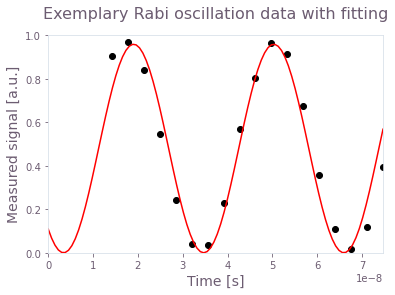The figure shows the result of a Rabi experiment for an amplitude $A_I = 0.2$ (circles) and the correponding cosine square fit (line).

You can now collect all the $I$ values from the previous experiment and establish the correspondence between $I$ and $A_I$. This is done in the cell below.

amplitude_interpolated_list = np.linspace(-0.2, 0.2, 100)
pulse_amp_array = np.concatenate((-pulse_amp_array[::-1], pulse_amp_array))
rabi_calibration_exp_I = np.concatenate(
(-np.asarray(rabi_calibration_exp_I[::-1]), np.asarray(rabi_calibration_exp_I))
)
f_amp_to_rabi = interpolate.interp1d(pulse_amp_array, rabi_calibration_exp_I)
rabi_interpolated_exp_I = f_amp_to_rabi(amplitude_interpolated_list)

f_rabi_to_amp = interpolate.interp1d(
rabi_interpolated_exp_I, amplitude_interpolated_list
)

plt.title("IBMQ Jakarta: Rabi rates calibration", fontsize=16, y=1.1)
plt.xlabel("Hardware input amplitude", fontsize=14)
plt.ylabel("Rabi rate [Hz]", fontsize=14)
plt.scatter(pulse_amp_array, rabi_calibration_exp_I)
plt.tick_params(axis="both", which="major", labelsize=14)
plt.plot(amplitude_interpolated_list, rabi_interpolated_exp_I)
plt.axvline(0, color="black", linestyle="dashed")
plt.axhline(0, color="black", linestyle="dashed")
plt.show()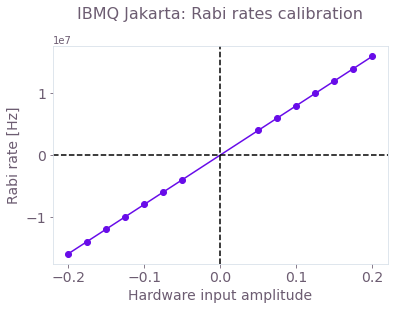Mapping between the Rabi rates and the dimensionless hardware input. The calibration was done in the interval $[-0.2, 0.2]$, where the relationship is linear.

### Verifying basic calibration

Now that you have performed the basic calibration, you may want to test it on your device. In the Application Note "Superconducting qubits: improving the performance of single qubit gates" you'll find a detailed explanation on how to construct robust pulses for superconducting qubit systems. Here, however, you don't need to create your own pulse as a previously generated control is provided to verify the calibration procedure. When executing the next cell, you'll import this control pulse, extract some of its properties for later use, and plot its values.

# Import pulses
gate_schemes = load_var(
"resources/superconducting-qubits-pulse-calibration/pulse_amplitude_robust"
)

# Extract pulse properties
number_of_segments = {}
duration_int = {}
duration = {}
segment_scale = {}
waveform = {}
for scheme_name, control in gate_schemes.items():
number_of_segments[scheme_name] = len(control["I"])
duration[scheme_name] = sum(values["duration"] for values in control["I"])
duration_int[scheme_name] = int(round(duration[scheme_name] / dt))
segment_scale[scheme_name] = (
duration_int[scheme_name] / number_of_segments[scheme_name]
)
I_values = [segment["value"] / 2 / np.pi for segment in control["I"]]
Q_values = [segment["value"] / 2 / np.pi for segment in control["Q"]]
A_I_values = np.repeat(f_rabi_to_amp(I_values), segment_scale[scheme_name])
A_Q_values = np.repeat(f_rabi_to_amp(Q_values), segment_scale[scheme_name])
waveform[scheme_name] = A_I_values + 1j * A_Q_values

# Plot pulses
for scheme_name, gate in gate_schemes.items():
print(scheme_name)
plot_controls(plt.figure(), gate)
plt.show()

Amplitude robust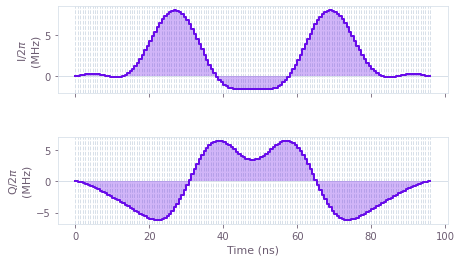I and Q components of the microwave drive on the qubit for an amplitude robust pulse that performs a $\pi/2$ rotation around the $x$ axis (an $X_{\pi/2}$ gate). Note that the values of the pulse waveform are given in Hz.

To verify the calibration, you can run this pulse on the device and check that it executes the desired gate. This is done in the next cell, where the outputs are the $(x, y, z)$ components of the Bloch vector for the qubit.

# Creating IBM schedules
ibm_evolution_times = {}
times_int = {}
pulse_evolution_program = {}
time_min = 64
time_step = 16
for scheme_name in gate_schemes.keys():
time_max = int(segment_scale[scheme_name] * number_of_segments[scheme_name])
times_int[scheme_name] = np.arange(time_min, time_max + time_step, time_step)
ibm_evolution_times[scheme_name] = times_int[scheme_name] * dt

if use_saved_data == False:
for scheme_name in gate_schemes.keys():
evolution_schedules = []
for meas_basis in bloch_basis:
for time_idx in times_int[scheme_name]:
schedule = pulse.Schedule(
name="Basis_%s_duration_%d" % (meas_basis, time_idx)
)
schedule += Play(Waveform(waveform[scheme_name][:time_idx]), drive_chan)
if meas_basis == "x":
schedule += inst_sched_map.get("u2", , P0=0.0, P1=np.pi)
schedule += measure_schedule << schedule.duration
if meas_basis == "y":
schedule += inst_sched_map.get("u2", , P0=0.0, P1=np.pi / 2)
schedule += measure_schedule << schedule.duration
if meas_basis == "z":
schedule += measure_schedule << schedule.duration
evolution_schedules.append(schedule)

pulse_evolution_program[scheme_name] = assemble(
evolution_schedules,
backend=backend,
meas_level=2,
meas_return="single",
shots=num_shots_per_point,
schedule_los=[{drive_chan: qubit_frequency_updated}]
* len(times_int[scheme_name])
* 3,
)

# Running the jobs
evolution_exp_results = {}
for scheme_name in gate_schemes.keys():
job = backend.run(pulse_evolution_program[scheme_name])
job_monitor(job)
evolution_exp_results[scheme_name] = job.result(timeout=120)

# Extract results
evolution_results_ibm = {}
for scheme_name in gate_schemes.keys():
evolution_basis = {}
for meas_basis in bloch_basis:
evolution_exp_data = np.zeros(len(times_int[scheme_name]))
for idx, time_idx in enumerate(times_int[scheme_name]):
counts = evolution_exp_results[scheme_name].get_counts(
"Basis_%s_duration_%d" % (meas_basis, time_idx)
)
excited_pop = 0
for bits, count in counts.items():
excited_pop += count if bits[::-1][qubit] == "1" else 0
evolution_exp_data[idx] = excited_pop / num_shots_per_point
evolution_basis[meas_basis] = evolution_exp_data
evolution_results_ibm[scheme_name] = evolution_basis

save_var(
"resources/superconducting-qubits-pulse-calibration/evolution_exp_results_jakarta_qubit_0",
evolution_results_ibm,
)
else:
evolution_results_ibm = load_var(
"resources/superconducting-qubits-pulse-calibration/evolution_exp_results_jakarta_qubit_0"
)


You can now use the BOULDER OPAL simulation feature to obtain the expected evolution of the qubit under the selected pulse and compare it with the experimental results.

# Q-CTRL simulations
simulated_bloch = {}
gate_times = {}
infidelities = {}
for scheme_name, control in gate_schemes.items():
print(scheme_name)
(
infidelities[scheme_name],
simulated_bloch[scheme_name],
gate_times[scheme_name],
) = simulation_coherent(control, 100)

# Plotting
gs = gridspec.GridSpec(1, len(gate_schemes.keys()), hspace=0.5)
fig = plt.figure()
fig.set_figheight(5)
fig.set_figwidth(8)
fig.suptitle(
"Time evolution under control pulses for qubit " + str(qubit), fontsize=16, y=1.05
)

for idx, scheme_name in enumerate(gate_schemes.keys()):
ax = fig.add_subplot(gs[idx])
for meas_basis in bloch_basis:
ax.plot(
gate_times[scheme_name],
simulated_bloch[scheme_name][meas_basis],
ls=lines[meas_basis],
label="%s: Q-CTRL simulation" % (meas_basis),
)
ax.scatter(
ibm_evolution_times[scheme_name],
1 - 2 * evolution_results_ibm[scheme_name][meas_basis],
label="%s: IBM experiments" % (meas_basis),
marker=markers[meas_basis],
)
ax.set_title(scheme_name, fontsize=14)
ax.set_xlabel("Time [s]", fontsize=14)
ax.set_ylabel("Bloch components", fontsize=14)
ax.tick_params(labelsize=14)
ax.legend(loc="best", bbox_to_anchor=(1, 1))

plt.show()

Amplitude robust

Your task calculate_coherent_simulation has completed.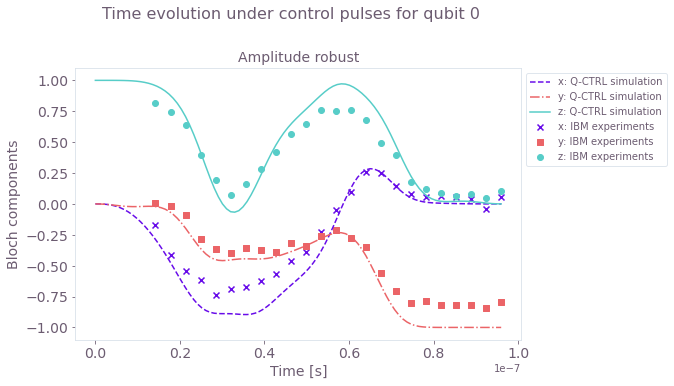Time evolution of the Bloch vector components for the amplitude-robust $X_{\pi/2}$ gate. The experimental results (symbols) follow the numerical simulation (lines) with deviations mostly due to readout errors.

## Fine-tuning of individual pulses

The basic calibration that you've just performed is general in the sense that it is independent of the pulse applied. However, it is not the most accurate calibration method. Note that, in a real device, the pulse that is programmed in your wave generator is most likely not what reaches the physical qubit due to hardware bandwidth limitations and imperfections in the control lines.

An improved calibration procedure starts from the basic calibration discussed before and introduces two scaling factors $S_{rel}$ and $S_{amp}$ so that

\begin{align*} \gamma(t) = S_{amp} (S_{rel} I(t) + i Q(t)). \end{align*}

The $S_{rel}$ factor allows you to account for relative differences between the designed and the actual values of $I$ and $Q$ reaching the qubit, while $S_{amp}$ accounts for an absolute amplitude scaling. Note that it is enough to scan these scaling factors around $1$ since $S_{amp} = S_{rel} = 1$ corresponds to the values obtained from the previous basic calibration.

For a procedure that is maximally sensitive to coherent errors due to miscalibration, you should apply your pulse multiple times to allow for these errors to pile up. For the $X_{\pi/2}$ pulses, you can use $N = (1 + 4n)$ repetitions with $n \in \{0, 1 \cdots \}$ so that the Bloch vector always ends up in the same point if the pulse is perfect. The calibration steps are:

1. Using two sequences with different numbers of pulse repetitions ($N_1$ and $N_2$), perform experiments where you scan over the values of $S_{rel}$ around $1$ (no scaling), and calculate the gate infidelity as a function of this scaling factor.

2. Find the value of the scaling factor, $S_{rel}$, that minimizes the infidelity for both repetition numbers. You could be tempted to use a single repetition number $N$ to speed up your calibration but this is not advisable. Note that, if you pick $N$ that is too small, there will be little variation of the infidelity with $S_{rel}$ and your calibration would be innacurate. If you select $N$ that is too large, you may see multiple infidelity minima as small changes in $S_{rel}$ can lead to full rotations in the Bloch sphere. In this case, you may be selecting the wrong scaling factor. Using two values allows you to find a single scaling that best calibrates your pulse.

3. Repeat step 2. but now scaning $S_{amp}$ and using the value of $S_{rel}$ found in the previous step. Again, find the scaling factor that minimizes the infidelith for both pulse repetition numbers.

### Relative scaling calibration

The next cell runs the scan over $S_{rel}$ described in step 1 for $N_1 = 49$ and $N_2 =101$ and then finds the best relative scaling described in step 2.

repetitions = np.array([49, 101])
scalings = np.linspace(0.95, 1.05, 50)

if use_saved_data == False:
pulse_program = {}
for scheme_name in gate_schemes.keys():
schedules = []
for meas_basis in bloch_basis:
for repetition in repetitions:
for scaling_factor_I in scalings:
Ival = np.real(waveform[scheme_name]) * scaling_factor_I
Qval = np.imag(waveform[scheme_name])
scaled_waveform = Ival + 1j * Qval
control_pulse = Play(Waveform(scaled_waveform), drive_chan)
schedule = pulse.Schedule(
name=f"pi_pulse_scal_{scaling_factor_I}_reps_"
+ str(repetition)
+ "_basis_"
+ str(meas_basis)
)
for reps in np.arange(repetition):
schedule += control_pulse
if meas_basis == "x":
schedule += inst_sched_map.get("u2", , P0=0.0, P1=np.pi)
schedule += measure_schedule << schedule.duration
if meas_basis == "y":
schedule += inst_sched_map.get("u2", , P0=0.0, P1=np.pi / 2)
schedule += measure_schedule << schedule.duration
if meas_basis == "z":
schedule |= measure_schedule << schedule.duration
schedules.append(schedule)
pulse_program[scheme_name] = assemble(
schedules,
backend=backend,
meas_level=2,
meas_return="single",
shots=num_shots_per_point,
schedule_los=[{drive_chan: qubit_frequency_updated}]
* (repetitions.shape)
* (scalings.shape)
* 3,
)

# Running the jobs
exp_results = {}
for scheme_name in gate_schemes.keys():
print("Control Scheme:", scheme_name)
job = backend.run(pulse_program[scheme_name])
print("Job id:", job.job_id())
job_monitor(job)
exp_results[scheme_name] = job.result(timeout=120)

# Extract results
I_calibration_data = {}
for scheme_name, results in exp_results.items():
print(scheme_name)
idx = 0
result_bloch = []
for meas_basis in bloch_basis:
full_res = []
for idx_rep, number_of_repetitions in enumerate(repetitions):
rep_res = []
for scaling_factor in scalings:
counts = results.get_counts()[idx]
excited_pop = 0
idx += 1
for bits, count in counts.items():
excited_pop += count if bits[::-1][qubit] == "1" else 0
rep_res.append(excited_pop / num_shots_per_point)
full_res.append(rep_res)
result_bloch.append(1 - 2 * np.asarray(full_res))
I_calibration_data[scheme_name] = np.asarray(result_bloch)

save_var(
"resources/superconducting-qubits-pulse-calibration/relative_calibration_data_jakarta_qubit_0",
I_calibration_data,
)

else:
I_calibration_data = load_var(
"resources/superconducting-qubits-pulse-calibration/relative_calibration_data_jakarta_qubit_0"
)

# Infidelities for repetition experiments
target_bloch = np.array([0, -1, 0])
bloch_infidelity = {}
for scheme_name in gate_schemes.keys():
bloch_vectors = np.array(
[
np.array(
[
I_calibration_data[scheme_name][:, rep, idx]
for idx in range(scalings.shape)
]
)
for rep in range(repetitions.shape)
]
)
bloch_infidelity[scheme_name] = (
1 - ((1 + np.dot(bloch_vectors, target_bloch)) / 2) ** 2
)

# Plotting
gs = gridspec.GridSpec(1, len(gate_schemes.keys()), wspace=0.2, hspace=0.4)
fig = plt.figure()
fig.set_figheight(5)
fig.set_figwidth(8)
fig.suptitle(
f"Relative scaling calibration from pulse repetitions", fontsize=16, y=1.02
)

best_scaling_I = {}
average_infidelity = {}
for idx, scheme_name in enumerate(gate_schemes.keys()):
ax = fig.add_subplot(gs[idx])
infidelity_smoothed = []
for idx, pulse_repetition in enumerate(repetitions):
infidelity_smoothed.append(
movingaverage(bloch_infidelity[scheme_name][idx, :], 3)
)
ax.set_title("Control: " + str(scheme_name), fontsize=14)
ax.scatter(
scalings,
bloch_infidelity[scheme_name][idx, :],
label="Infidelity data for %s pulse repetitions" % (pulse_repetition),
)
ax.plot(
scalings[1:-1],
infidelity_smoothed[-1],
label="Smoothed infidelity for %s pulse repetitions" % (pulse_repetition),
)
ax.set_xlabel("Relative scaling", fontsize=14)
ax.set_ylabel("Infidelity", fontsize=14)
ax.tick_params(labelsize=14)
average_infidelity[scheme_name] = np.average(infidelity_smoothed, axis=0)
best_scaling_I[scheme_name] = scalings[
np.argmin(average_infidelity[scheme_name]) + 1
]
ax.plot(
scalings[1:-1],
average_infidelity[scheme_name],
label="Average infidelity",
c="k",
)
ax.axvline(best_scaling_I[scheme_name], ls="--")
ax.text(
scalings[np.argmin(infidelity_smoothed) + 1],
np.max(infidelity_smoothed),
"Best $S_{rel}$=" + str(round(scalings[np.argmin(infidelity_smoothed) + 1], 3)),
fontsize=14,
)
ax.set_ylim(0, 1.05)
ax.legend(loc="best", bbox_to_anchor=(1, 1))
plt.show()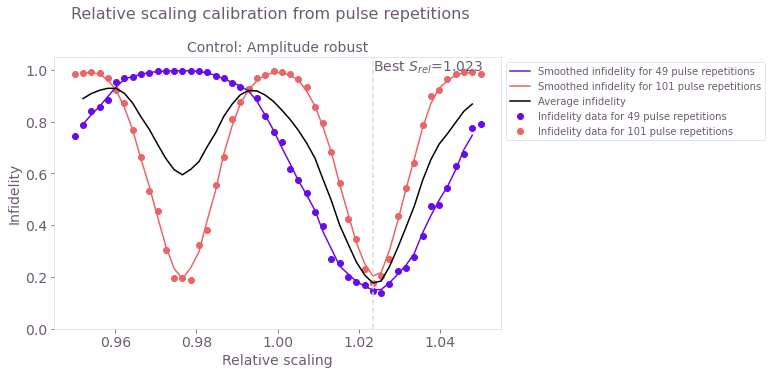Infidelity as a function of $S_{rel}$ for 49 and 101 repetitions of the pulse. The solid lines are a moving average of the experimental results (symbols) using the values from 3 points. The black solid line is the average between the 49 and 101 repetitions experiments. The scaling factor that you should use when calibrating your pulse is the one that minimizes the average infidelity.

### Amplitude scaling calibration

The next cell repeats steps 1 and 2 but now for the scaling factor $S_{amp}$.

repetitions = np.array([49, 101])
scalings = np.linspace(0.95, 1.05, 50)

if use_saved_data == False:
pulse_program = {}
for scheme_name in gate_schemes.keys():
schedules = []
for meas_basis in bloch_basis:
for repetition in repetitions:
for scaling_factor in scalings:
scaled_waveform = (
np.real(waveform[scheme_name]) * best_scaling_I[scheme_name]
+ 1j * np.imag(waveform[scheme_name])
) * scaling_factor
control_pulse = Play(Waveform(scaled_waveform), drive_chan)
schedule = pulse.Schedule(
name=f"pi_pulse_scal_{scaling_factor}_reps_"
+ str(repetition)
+ "_basis_"
+ str(meas_basis)
)
for reps in np.arange(repetition):
schedule += control_pulse
if meas_basis == "x":
schedule += inst_sched_map.get("u2", , P0=0.0, P1=np.pi)
schedule += measure_schedule << schedule.duration
if meas_basis == "y":
schedule += inst_sched_map.get("u2", , P0=0.0, P1=np.pi / 2)
schedule += measure_schedule << schedule.duration
if meas_basis == "z":
schedule |= measure_schedule << schedule.duration
schedules.append(schedule)
pulse_program[scheme_name] = assemble(
schedules,
backend=backend,
meas_level=2,
meas_return="single",
shots=num_shots_per_point,
schedule_los=[{drive_chan: qubit_frequency_updated}]
* (repetitions.shape)
* (scalings.shape)
* 3,
)

# Running the jobs
exp_results = {}
for scheme_name in gate_schemes.keys():
print("Control Scheme:", scheme_name)
job = backend.run(pulse_program[scheme_name])
print("Job id:", job.job_id())
job_monitor(job)
exp_results[scheme_name] = job.result(timeout=120)

# Extract results
amplitude_calibration_data = {}
for scheme_name, results in exp_results.items():
print(scheme_name)
idx = 0
result_bloch = []
for meas_basis in bloch_basis:
full_res = []
for idx_rep, number_of_repetitions in enumerate(repetitions):
rep_res = []
for scaling_factor in scalings:
counts = results.get_counts()[idx]
excited_pop = 0
idx += 1
for bits, count in counts.items():
excited_pop += count if bits[::-1][qubit] == "1" else 0
rep_res.append(excited_pop / num_shots_per_point)
full_res.append(rep_res)
result_bloch.append(1 - 2 * np.asarray(full_res))
amplitude_calibration_data[scheme_name] = np.asarray(result_bloch)

save_var(
"resources/superconducting-qubits-pulse-calibration/amplitude_calibration_data_jakarta_qubit_0",
amplitude_calibration_data,
)
else:
amplitude_calibration_data = load_var(
"resources/superconducting-qubits-pulse-calibration/amplitude_calibration_data_jakarta_qubit_0"
)

# Infidelities for repetition experiments
bloch_infidelity = {}
for scheme_name in gate_schemes.keys():
bloch_vectors = np.array(
[
np.array(
[
amplitude_calibration_data[scheme_name][:, rep, idx]
for idx in range(scalings.shape)
]
)
for rep in range(repetitions.shape)
]
)
bloch_infidelity[scheme_name] = (
1 - ((1 + np.dot(bloch_vectors, target_bloch)) / 2) ** 2
)

gs = gridspec.GridSpec(1, len(gate_schemes.keys()))
fig = plt.figure()
fig.set_figheight(5)
fig.set_figwidth(8)
fig.suptitle(
f"Amplitude scaling calibration from pulse repetitions", fontsize=16, y=1.02
)

best_scaling_amplitude = {}
average_infidelity = {}
for idx, scheme_name in enumerate(gate_schemes.keys()):
ax = fig.add_subplot(gs[idx])
infidelity_smoothed = []
for idx, pulse_repetition in enumerate(repetitions):
infidelity_smoothed.append(
movingaverage(bloch_infidelity[scheme_name][idx, :], 5)
)
ax.set_title("Control: " + str(scheme_name), fontsize=14)
ax.scatter(
scalings,
bloch_infidelity[scheme_name][idx, :],
label="Infidelity data for %s pulse repetitions" % (pulse_repetition),
)
ax.plot(
scalings[2:-2],
infidelity_smoothed[-1],
label="Smoothed infidelity for %s pulse repetitions" % (pulse_repetition),
)
ax.set_xlabel("Amplitude scaling", fontsize=14)
ax.set_ylabel("Infidelity", fontsize=14)
ax.tick_params(labelsize=14)
average_infidelity[scheme_name] = np.average(infidelity_smoothed, axis=0)
best_scaling_amplitude[scheme_name] = scalings[
np.argmin(average_infidelity[scheme_name]) + 2
]
ax.plot(
scalings[2:-2],
average_infidelity[scheme_name],
label="Average infidelity",
c="k",
)
ax.text(
best_scaling_amplitude[scheme_name],
0.35,  # np.max(infidelity_smoothed[-1]),
"Best $S_{amp}$:" + str(round(best_scaling_amplitude[scheme_name], 3)),
fontsize=14,
)
ax.axvline(best_scaling_amplitude[scheme_name], ls="--")
ax.set_ylim(0, 0.5)
ax.legend(loc="best", bbox_to_anchor=(1, 1))
plt.show()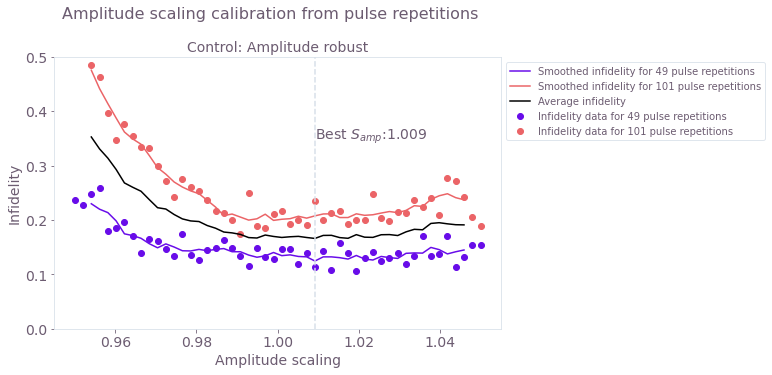Infidelity as a function of the amplitude scaling factor $S_{amp}$. As in the previous step, the solid lines are a moving average of the experimental results (symbols), and the black solid line is the average between the two smoothed curves. In this figure, you can see that this particular pulse is mostly insensitive to the overall scaling factor as it has been designed to be robust against amplitude noise (check the Application Note "Superconducting qubits: improving the performance of single qubit gates" for more information on the design of robust pulses).

## Calibration with automated optimization

An alternative way of calibrating your pulse is to use BOULDER OPAL's closed-loop optimization tools to find the calibration parameters automatically. In each iteration of the procedure, the optimizer will pick a set of calibration parameters, transform the pulse, send it to the device, and receive back the measured infidelity. As more iterations are performed, the optimizable parameters will converge to the values that minimize the infidelity.

You will use the same pulse parametrization as before with

\begin{align*} \gamma(t) = S_{amp} (S_{rel} A_I(t) + i A_Q(t)), \end{align*}

and set $S_{amp}$ and $S_{rel}$ as the variables for the automated optimization. Since the initial Rabi rate calibration has been already performed for this device, you can restrict your search for values of $S_{amp}$ and $S_{rel}$ around one. In what follows, the optimization bounds are set to be $S_{amp} \in [0.8,1.2]$ and $S_{rel} \in [0.8,1.2]$, that is, within $20 \%$ of the values of the initial rough Rabi calibration.

In each iteration of the calibration loop, you'll need to send a pulse to the device. The functions in the cell below show how to do this using Qiskit. If you want to deploy this calibration in your own experiment, you'll need to modify these functions accordingly.

error_mitigation = True

def run_ibm_experiments(control, calibration_pairs, repetitions, qubit):

I_values = np.array([segment["value"] for segment in control["I"]])
Q_values = np.array([segment["value"] for segment in control["Q"]])

schedules = []
for scal in calibration_pairs:
scaled_waveform = (I_values * scal + 1j * Q_values) * scal
control_pulse = Play(Waveform(scaled_waveform), DriveChannel(qubit))
for meas_basis in bloch_basis:
for repetition in repetitions:
schedule = pulse.Schedule(
name=f"pulse_scal_{scal}_reps_"
+ str(repetition)
+ "_basis_"
+ str(meas_basis)
)
for reps in np.arange(repetition):
schedule += control_pulse
if meas_basis == "x":
schedule += inst_sched_map.get("u2", [qubit], P0=0.0, P1=np.pi)
schedule += measure_schedule << schedule.duration
if meas_basis == "y":
schedule += inst_sched_map.get("u2", [qubit], P0=0.0, P1=np.pi / 2)
schedule += measure_schedule << schedule.duration
if meas_basis == "z":
schedule |= measure_schedule << schedule.duration
schedules.append(schedule)

# Running the jobs
job = execute(schedules, backend, shots=num_shots_per_point)
print("Job id:", job.job_id())
job_monitor(job)
exp_results = job.result(timeout=120)

return exp_results

def extract_results(results, calibration_pairs):

idx = 0
pairs_results = []
if mitigation:
results = measurementFilter.apply(results)

for scaling_pair in calibration_pairs:
basis_results = []
for meas_basis in bloch_basis:
rep_results = []
for number_of_repetitions in repetitions:
count = marginal_counts(results.get_counts()[idx], meas_qubits=)
excited_pop = 0
idx += 1
excited_pop += count["1"]
rep_results.append(excited_pop / num_shots_per_point)
basis_results.append(rep_results)
pairs_results.append(1 - 2 * np.asarray(basis_results))
calibration_data = np.asarray(pairs_results)

# Infidelities for repetition experiments
target_bloch = np.array([0, -1, 0])
bloch_vectors = np.array(
[
np.array([calibration_data[idx, :, rep] for rep in range(len(repetitions))])
for idx in range(len(calibration_pairs))
]
)
bloch_infidelity = 1 - ((1 + np.dot(bloch_vectors, target_bloch)) / 2) ** 2

cost_infidelity = np.average(bloch_infidelity, axis=1)
print("Best cost in this step:", np.min(cost_infidelity))

return cost_infidelity

def conductErrorMitigation(shots):
"""Measure the state preparation and measurement error"""

cal_circuits, state_labels = qs.ignis.mitigation.measurement.complete_meas_cal(
qubit_list=np.arange(7), circlabel="MeasErrMitCal"
)

cal_job = qs.execute(cal_circuits, backend=backend, shots=shots)
qs.tools.monitor.job_monitor(cal_job)
cal_results = cal_job.result()

meas_fitter = qs.ignis.mitigation.measurement.CompleteMeasFitter(
cal_results, state_labels
)

# Set the object's measurementFilter here
meas_filter = meas_fitter.filter

ErrorMitigationComplete = True
return meas_filter

if use_saved_data == False and error_mitigation:
SPAMshots = 1024 * 4
measurementFilter = conductErrorMitigation(shots=SPAMshots)


Here, you'll recalibrate the control pulse imported at the beginning of this notebook. This pulse has already been transformed by the initial Rabi calibration with the result stored in the waveform['Amplitude robust'] array.

pulse_to_calibrate = {
"I": [
{"duration": d, "value": v}
for d, v in zip(
dt * np.ones(waveform["Amplitude robust"].shape),
np.real(waveform["Amplitude robust"]),
)
],
"Q": [
{"duration": d, "value": v}
for d, v in zip(
dt * np.ones(waveform["Amplitude robust"].shape),
np.imag(waveform["Amplitude robust"]),
)
],
}


The next cell sets up the automated optimization and runs the calibration loop. Note that in the previous procedure you had to carefully and manually pick two different pulse repetitions for the calibration analysis. Now you will directly set the optimization cost as the average infidelity for multiple repetitions (4 in the case below), streamlining the calibration process by requiring less human intervention.

To minimize the number of API calls to the IBM Quantum device, the calibration procedure will make use of the batching feature of the Q-CTRL Python package (see this User guide for more information). By setting test_point_count to 5, you'll send five independent jobs under a single request in the queue. In this way, the optimizer will use the result from five different sets of calibration parameters in each iteration.

if use_saved_data:
best_cost_array = load_var(
"resources/superconducting-qubits-pulse-calibration/best_cost_array_jakarta_June_8"
)
best_param_array = load_var(
"resources/superconducting-qubits-pulse-calibration/best_control_array_jakarta_June_8"
)
print(f"Infidelity: {best_cost_array[-1]}")
print(f"Calibration parameters: {best_param_array[-1]}")
else:
repetitions = np.arange(5, 42, 12)
relative_bounds = (0.8, 1.2)
amplitude_bounds = (0.8, 1.2)
test_point_count = 5

calibration_result = {}
best_cost_array = []
best_param_array = []

# Create random pairs for initial batch
rng = np.random.default_rng()
parameter_set = [
[
rng.uniform(relative_bounds, relative_bounds),
rng.uniform(amplitude_bounds, amplitude_bounds),
]
for idx in np.arange(test_point_count)
]

# Run the experiment with the initial random calibration parameters
exp_results = run_ibm_experiments(
pulse_to_calibrate, parameter_set, repetitions, qubit
)
cost_results = extract_results(exp_results, parameter_set)

# OPTIMIZATION SETUP
# Define initialization object for the automated closed-loop optimization
bound = qctrl.types.closed_loop_optimization_step.BoxConstraint(
lower_bound=relative_bounds,
upper_bound=relative_bounds,
)
bound_2 = qctrl.types.closed_loop_optimization_step.BoxConstraint(
lower_bound=amplitude_bounds,
upper_bound=amplitude_bounds,
)
initializer_GP = (
qctrl.types.closed_loop_optimization_step.GaussianProcessInitializer(
bounds=[bound, bound_2],
rng_seed=0,
)
)

# Define state object for the closed-loop optimization
optimizer = qctrl.types.closed_loop_optimization_step.Optimizer(
gaussian_process_initializer=initializer_GP,
)

best_cost, best_controls = min(
zip(cost_results, parameter_set), key=lambda params: params
)
optimization_count = 0

best_cost_array.append(best_cost)
best_param_array.append(best_controls)

# Run the optimization loop until the cost is sufficiently small or when it reaches 10 steps
idx = 0
while best_cost > 0.005 and idx <= 10:
# Print the current best cost.
optimization_steps = (
"optimization step" if optimization_count == 1 else "optimization steps"
)
print(
f"Best infidelity after {optimization_count} BOULDER OPAL {optimization_steps}: {best_cost}"
)

# Organize the experiment results into the proper input format
results = [
qctrl.types.closed_loop_optimization_step.CostFunctionResult(
parameters=list(parameters),
cost=cost,
cost_uncertainty=0.1,
)
for parameters, cost in zip(parameter_set, cost_results)
]

# Call the automated closed-loop optimizer and obtain the next set of test points
optimization_result = qctrl.functions.calculate_closed_loop_optimization_step(
optimizer=optimizer,
results=results,
test_point_count=test_point_count,
)
optimization_count += 1

# Organize the data returned by the automated closed-loop optimizer
parameter_set = np.array(
[test_point.parameters for test_point in optimization_result.test_points]
)
optimizer = qctrl.types.closed_loop_optimization_step.Optimizer(
state=optimization_result.state
)

# Obtain experiment results that the automated closed-loop optimizer requested
exp_results = run_ibm_experiments(
pulse_to_calibrate, parameter_set, repetitions, qubit
)
cost_results = extract_results(exp_results, parameter_set)

params_array.append(parameter_set)

# Record the best results after this round of experiments
cost, controls = min(
zip(cost_results, parameter_set), key=lambda params: params
)

if cost < best_cost:
best_cost = cost
best_controls = controls
idx += 1
best_cost_array.append(best_cost)
best_param_array.append(best_controls)
print("Best parameters:", best_controls)

# Print final best cost.
print(f"Infidelity: {best_cost}")
print(f"Calibration parameters: {best_controls}")
calibration_result[qubit] = best_controls

Infidelity: 0.00827659524514815
Calibration parameters: [1.02161937 1.04297263]

# Plotting
gs = gridspec.GridSpec(1, 1)
fig = plt.figure()
fig.set_figheight(5)
fig.set_figwidth(8)

ax = fig.add_subplot(gs)
ax.set_title("Convergence of automated pulse calibration", fontsize=16)
ax.scatter(np.arange(12), best_cost_array)
ax.plot(best_cost_array)
ax.set_xlabel("Optimization step", fontsize=14)
ax.set_ylabel("Cost (average infidelity)", fontsize=14)
ax.tick_params(labelsize=14)

plt.show()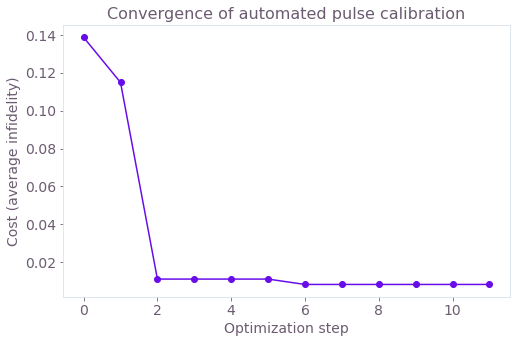Gate infidelity averaged for multiple repetitions of the pulses (5, 17, 29, and 41, in this case) as a function of the number of optimization steps. The calibration converges after a couple of steps, with the cost below $1\%$, after readout error mitigation. The final relative calibration parameter is $S_{rel} = 1.022$, which is close to the value $1.023$ found with the previous scheme. For the amplitude scaling, the automated calibration gave $S_{amp} = 1.043$, while the previous result was $1.009$. Note that you started with a pulse that is robust to amplitude noise and, therefore, less sensitive to variations in $S_{amp}$ by design.

## Verifying calibration using a pulse repetition sequence

Now that you have found the scaling factors that calibrate your particular pulse, you can verify it by performing a new pulse repetition experiment.

repetitions = np.arange(1, 151, 4)
num_shots_per_point = 2048
calibration_results = {
"Basic Rabi calibration": [1, 1],
"Fine-tuned calibration": [
best_scaling_I["Amplitude robust"],
best_scaling_amplitude["Amplitude robust"],
],
"Automated calibration": best_param_array[-1],
}

if use_saved_data == False:
# Full pulse calibration
calibrated_waveform = {}
for scheme_name, scalings in calibration_results.items():
calibrated_waveform[scheme_name] = (
scalings * np.real(waveform["Amplitude robust"])
+ 1j * np.imag(waveform["Amplitude robust"])
) * scalings

pulse_program = {}
for scheme_name, calibrated_pulse in calibrated_waveform.items():
schedules = []
for meas_basis in bloch_basis:
for repetition in repetitions:
control_pulse = Play(Waveform(calibrated_pulse), drive_chan)
schedule = pulse.Schedule(
name=f"pulse_reps_" + str(repetition) + "_basis_" + str(meas_basis)
)
for reps in np.arange(repetition):
schedule += control_pulse
if meas_basis == "x":
schedule += inst_sched_map.get("u2", , P0=0.0, P1=np.pi)
schedule += measure_schedule << schedule.duration
if meas_basis == "y":
schedule += inst_sched_map.get("u2", , P0=0.0, P1=np.pi / 2)
schedule += measure_schedule << schedule.duration
if meas_basis == "z":
schedule |= measure_schedule << schedule.duration
schedules.append(schedule)
pulse_program[scheme_name] = assemble(
schedules,
backend=backend,
meas_level=2,
meas_return="single",
shots=num_shots_per_point,
schedule_los=[{drive_chan: qubit_frequency_updated}]
* (repetitions.shape)
* 3,
)

# Running the jobs
exp_results = {}
for scheme_name, program in pulse_program.items():
print("Control Scheme:", scheme_name)
job = backend.run(program)
print("Job id:", job.job_id())
job_monitor(job)
exp_results[scheme_name] = job.result(timeout=120)

# Extract results
calibrated_pulse_performance = {}
for scheme_name, results in exp_results.items():
print(scheme_name)
idx = 0
result_bloch = []
for meas_basis in bloch_basis:
full_res = []
for idx_rep, number_of_repetitions in enumerate(repetitions):
rep_res = []
counts = results.get_counts()[idx]
excited_pop = 0
for bits, count in counts.items():
excited_pop += count if bits[::-1][qubit] == "1" else 0
rep_res.append(excited_pop / num_shots_per_point)
full_res.append(rep_res)
idx += 1
result_bloch.append(1 - 2 * np.asarray(full_res))
calibrated_pulse_performance[scheme_name] = np.asarray(result_bloch)

save_var(
"resources/superconducting-qubits-pulse-calibration/calibrated_pulse_performance_jakarta_qubit_0",
calibrated_pulse_performance,
)
else:
calibrated_pulse_performance = load_var(
"resources/superconducting-qubits-pulse-calibration/calibrated_pulse_performance_jakarta_qubit_0"
)

# Infidelities for repetition experiments
bloch_infidelity = {}
for scheme_name, performance in calibrated_pulse_performance.items():
bloch_vectors = performance[:, :, 0].T
bloch_infidelity[scheme_name] = (
1 - ((1 + np.dot(bloch_vectors, target_bloch)) / 2) ** 2
)

# Plotting
gs = gridspec.GridSpec(1, 1)
fig = plt.figure()
fig.set_figheight(5)
fig.set_figwidth(8)
fig.suptitle(f"Pulse performance after calibration", fontsize=16, y=0.95)

ax = fig.add_subplot(gs)
for scheme_name, infidelity in bloch_infidelity.items():
ax.plot(repetitions, infidelity, marker="o", label=scheme_name)
ax.set_xlabel("Pulse repetition number", fontsize=14)
ax.set_ylabel("Infidelity", fontsize=14)
ax.tick_params(labelsize=14)
ax.set_ylim(0, 1.05)
ax.legend(loc="best")
plt.show()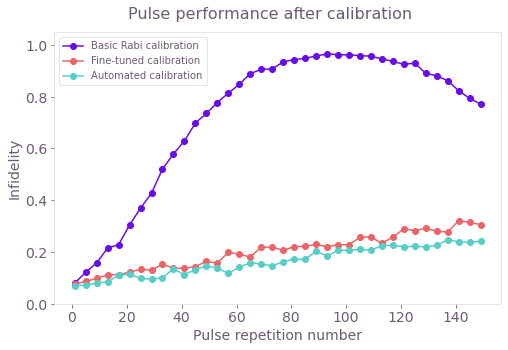Gate performance under a pulse repetition experiment. Both the fine-tuned and the automated calibrations of the pulse correct the accumulation of errors that quickly build up when using just the basic Rabi calibration.